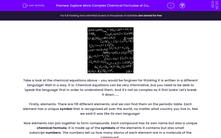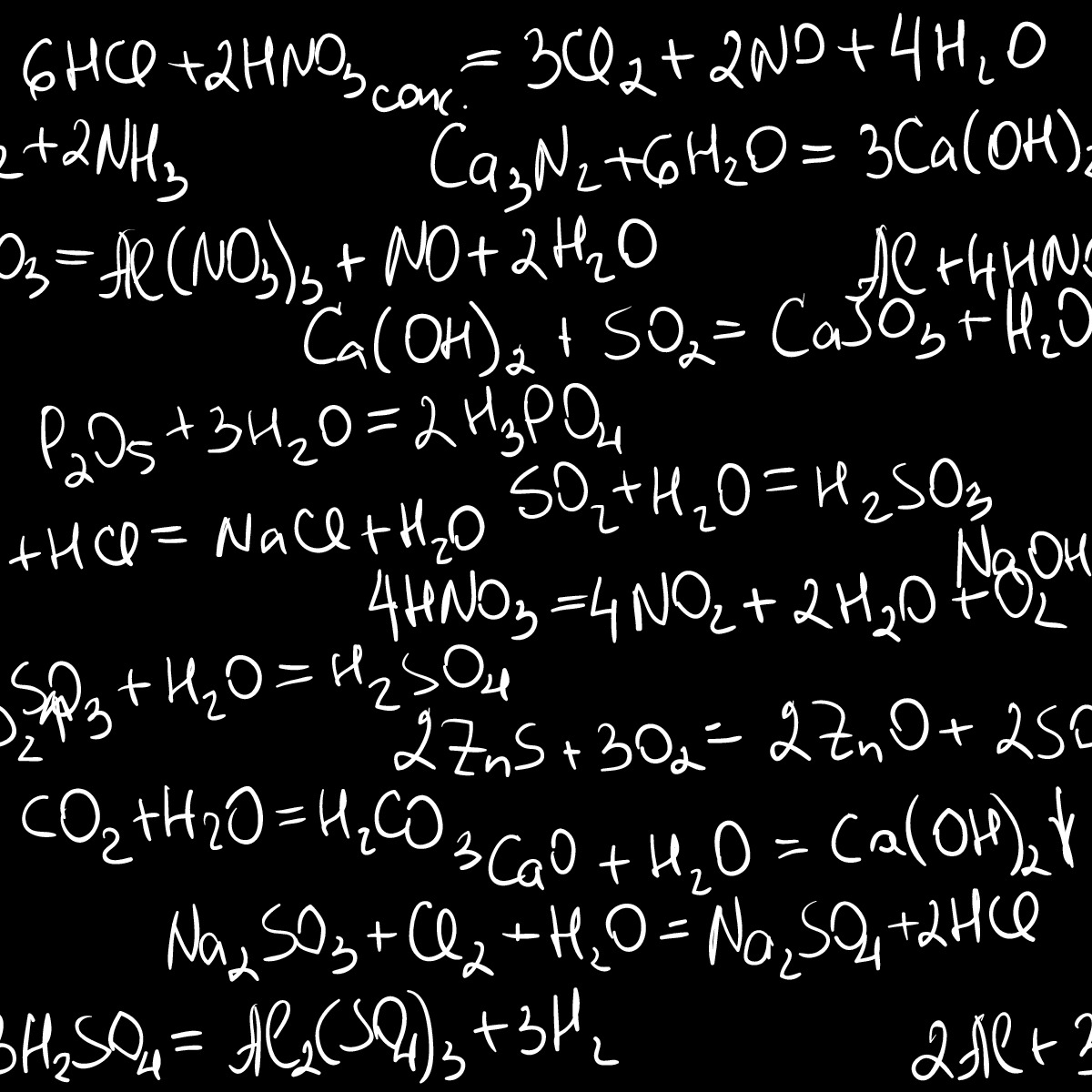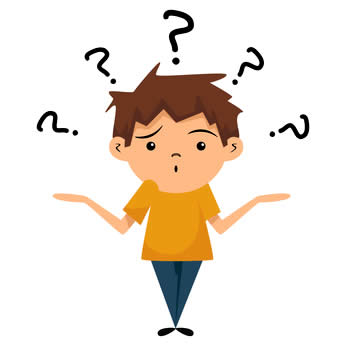# Explore More Complex Chemical Formulae of Compounds

In this worksheet, students will explore the chemical formulae of compounds.Key stage:  KS 3

Curriculum topic:   Chemistry: Atoms, Elements and Compounds

Curriculum subtopic:   Chemical Symbols and Formulae

Difficulty level:#### Worksheet OverviewTake a look at the chemical equations above - you would be forgiven for thinking it is written in a different language! Well in a way, it is. Chemical equations can be very informative, but you need to be able to 'speak the language' first in order to understand them. And it's not as complex as it first looks! Let's break it down......

Firstly, elements. There are 118 different elements, and we can find them on the periodic table. Each element has a unique symbol that is recognised all over the world, no matter what country you live in. See we said it was like its own language!

Now elements can join together to form compounds. Each compound has its own name but also a unique chemical formula. It is made up of the symbols of the elements it contains but also small subscript numbers. The numbers tell us how many atoms of each element are in a molecule of the compound.

For example H2SO4 contains three elements, H (hydrogen), S (sulfur) and O (oxygen). The numbers tell us how many of each atom there are in a molecule of the compound, so in this case, as there is a small 2 after H it tells us there are two atoms of hydrogen. There is no number after S which tells us there is only one atom present. There is a little 4 after O, so that tells us that there are four atoms of oxygen in a molecule of the compound.

By the way, the word 'formulae' is the plural of the word formula. If we're talking about more than one formula, we usually say formulae.

Now sometimes in a chemical equation you may see a large number before a symbol. For example 4NO2. Now that large 4 means '4 molecules of nitrogen dioxide (NO2) so in terms of working out how many atoms of each element are in this many molecules of the compound, we just have to multiply everything by 4. So there are 4 atoms of N (nitrogen) and 8 atoms of O (oxygen)!Don't worry if you feel like the boy above!! That's a lot to take in - learning a new 'language' requires practice, so let's have a go at some questions.

### What is EdPlace?

We're your National Curriculum aligned online education content provider helping each child succeed in English, maths and science from year 1 to GCSE. With an EdPlace account you’ll be able to track and measure progress, helping each child achieve their best. We build confidence and attainment by personalising each child’s learning at a level that suits them.

Get started••••